# What is Capacitance, Capacitor Series and Parallel Connection

## Capacitance:

The measured value of capacitor is called capacitance. The exact definition is the ability of a capacitor to store electric charge is measured. It is same like in resistor’s value as resistance, inductor value as inductance and capacitor value as capacitance.

Capacitance can be mathematically expressed by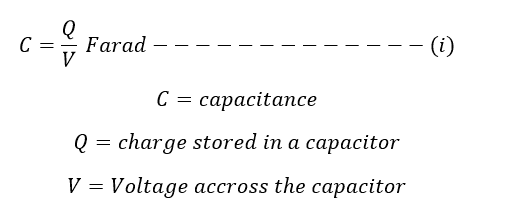Also, it is defined as the ratio of charge stored by capacitor to voltage V across the same capacitor.

Note: When the voltage across the capacitor or the capacitor voltage reaches or equal to source voltage means capacitor does not charge. No charge flows.

The unit of capacitance is Farad (F). To Honor Sir Michal Faraday (the inventor of most popular electrical law of electromagnetic induction), the unit of capacitor is named in Farad. Actually, one Farad is very large unit which means the size of the capacitor comes very bigger and most capacitors are rated in micro Farad (uF= 1 x 10-6) or micro farad (pF) or Pico Farad (pF) 1 pF = 1 x 10-12F

If Q=1 coulomb and V=1 volt, then capacitance is 1F. That’s we can say that 1 Farad is equal to 1 Coulombs/1volt.

One Farad is defined as the capacitance of a capacitor between the plates of which there appears a potential difference of I volt when it is charged by 1 coulomb of electricity.

### How to calculate capacitance value for two parallel plates capacitor: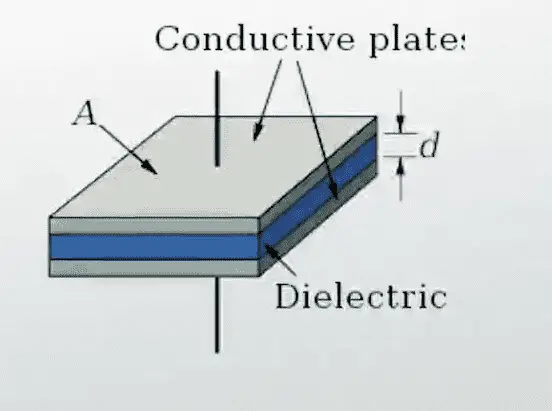Let us consider a parallel plate capacitor in which the upper and lower plates are separated by some distance of d meters. There is a potential difference of V volts between the two plates, therefore work required in transferring coulomb of charge from one plate to another is V Joules; since the work is the product of force and distance d the force experienced by the charge is the electric field strength E is given by ..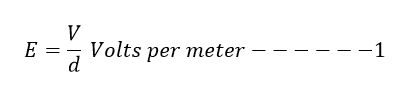The electric flux density D is given by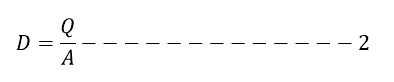The relation between electric flux density and electric field in terminals is given by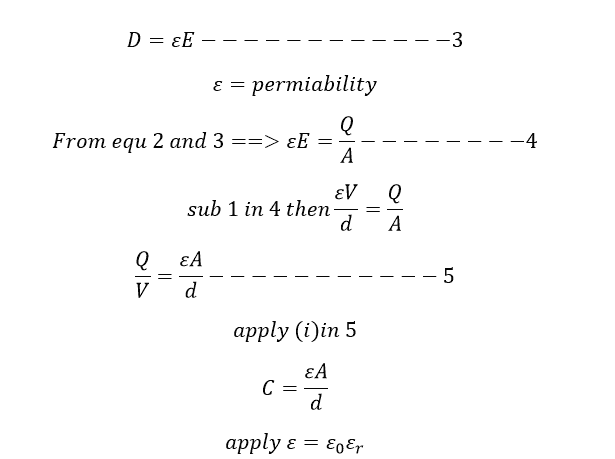The parallel plate capacitor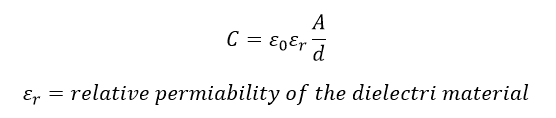Here the relative permeability of the material vary according to the type of dielectric material is used to construct a capacitor.

### Energy stored in a capacitor:

Capacitor stores energy in the form of electric field. Work has to be done in transferring the charge from one plate to another. Let the potential difference between the plates be V and the charge on the capacitor be q. Then, the work done in moving coulomb of charge from one plate to another plate is V. If dq is the additional charge transferred to the plate, then work done is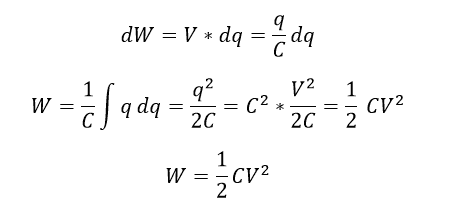### Capacitors in series connection: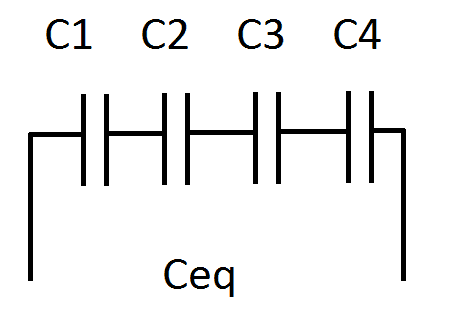If you connect four capacitors C1,C2,C3 and C4 in series connection…the resultant capacitance value is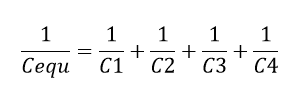The reciprocal of equivalent capacitance value is equal to sum of all reciprocal of individual capacitance value .It is opposite of inductance and resistance are in series connection.

### Key Points:

If you connect capacitor in series the resultant capacitance value comes lesser than the previous value. If you want to reduce the total capacitance value you can add a capacitor in series connection with existing.

### Capacitor in parallel connection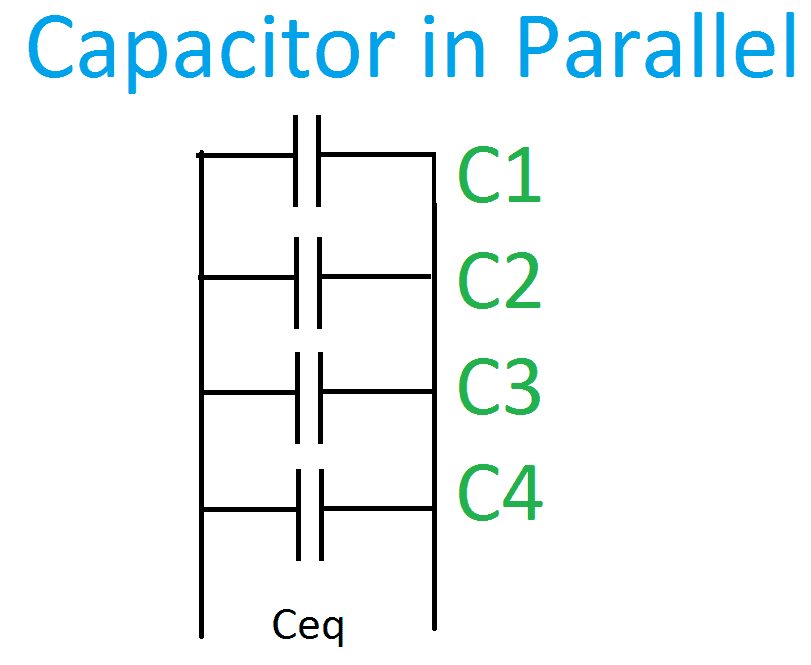If you connect four capacitors C1, C2, C3 and C4 in parallel connection…the resultant capacitance value is..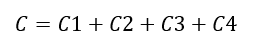The resultant capacitance is equal to sum of individual capacitance value.

### Key Points:

It is opposite of inductance and resistance are in series connection.

To increase the circuit’s capacitance value, you have to add a capacitor in parallel with existing circuit.